Electrical CircuitsPage 1

WATCH ALL SLIDES

Slide 1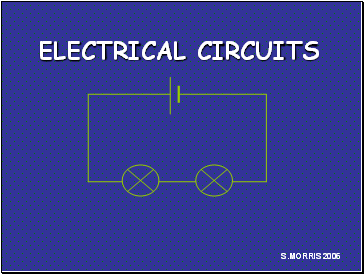S.MORRIS 2006

ELECTRICAL CIRCUITS

Slide 2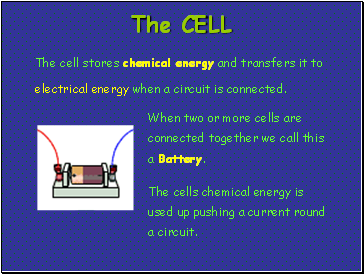The Cell

The cell stores chemical energy and transfers it to electrical energy when a circuit is connected.

When two or more cells are connected together we call this a Battery.

The cells chemical energy is used up pushing a current round a circuit.

Slide 3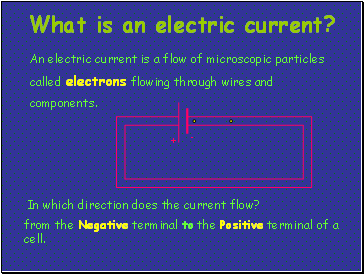What is an electric current?

An electric current is a flow of microscopic particles called electrons flowing through wires and components.

+

-

In which direction does the current flow?

from the Negative terminal to the Positive terminal of a cell.

Slide 4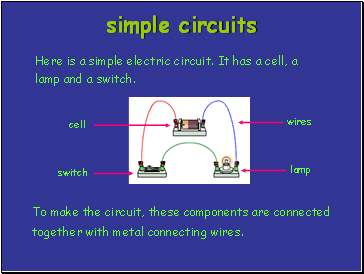Simple circuits

Here is a simple electric circuit. It has a cell, a lamp and a switch.

To make the circuit, these components are connected together with metal connecting wires.

cell

lamp

switch

wires

Slide 5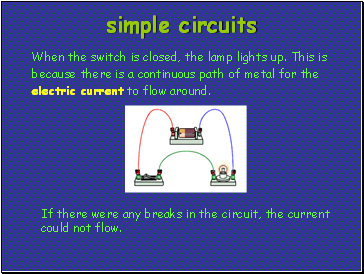simple circuits

When the switch is closed, the lamp lights up. This is because there is a continuous path of metal for the electric current to flow around.

If there were any breaks in the circuit, the current could not flow.

Slide 6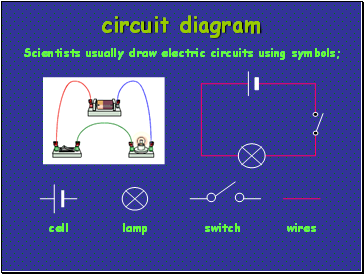Circuit diagram

cell

switch

lamp

wires

Scientists usually draw electric circuits using symbols;

Slide 7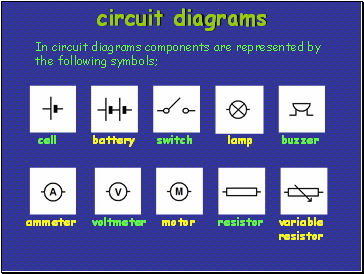circuit diagrams

In circuit diagrams components are represented by the following symbols;

cell

battery

switch

lamp

motor

ammeter

voltmeter

buzzer

resistor

variable resistor

Slide 8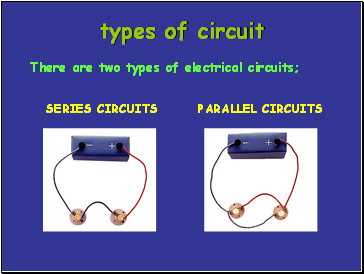Types of circuit

There are two types of electrical circuits;

SERIES CIRCUITS

PARALLEL CIRCUITS

Slide 9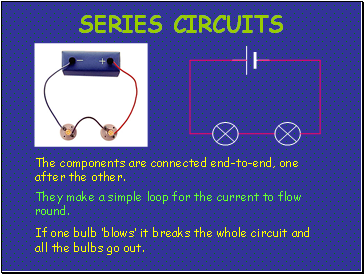Series circuits

The components are connected end-to-end, one after the other.

They make a simple loop for the current to flow round.

If one bulb ‘blows’ it breaks the whole circuit and all the bulbs go out.

Slide 10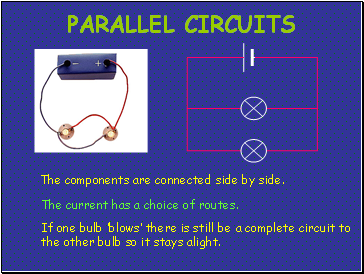Parallel circuits

The current has a choice of routes.

The components are connected side by side.

If one bulb ‘blows’ there is still be a complete circuit to the other bulb so it stays alight.

Slide 11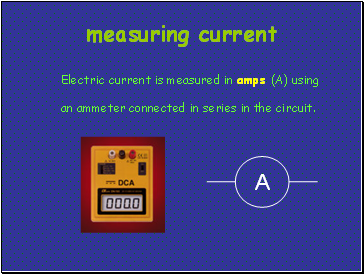Go to page:
1  2  3